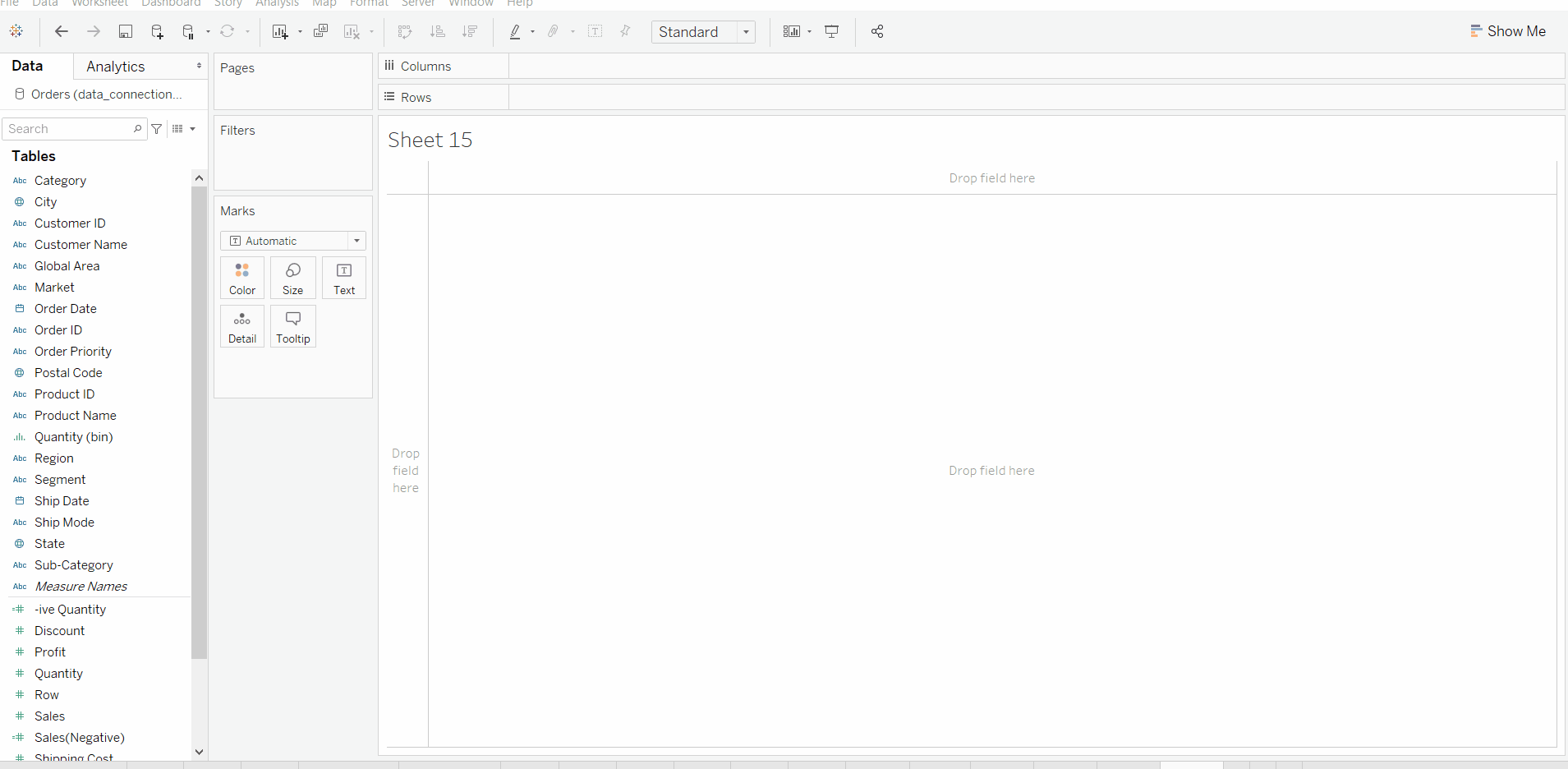Open In App
Related Articles
• Write an Interview Experience
• Tableau Tutorial

# Logical Functions in Tableau

In this article, we will learn about logical functions and it’s used in Tableau. For this first look into two terms :

• Tableau: Tableau is a very powerful data visualization tool that can be used by data analysts, scientists, statisticians, etc. to visualize the data and get a clear opinion based on the data analysis. Tableau is very famous as it can take in data and produce the required data visualization output in a very short time.
• Logical Function: Tableau provides various Logical Functions to perform logical operations on our data. They are Tableau AND, NOT, OR, IF, ELSEIF, IF Else, CASE, ISNULL, IFNULL, ZN, IIF, etc.

Let’s discuss every logical function one by one with an example. Dataset used in the given examples is Dataset.

AND Function: The AND Function is employed to see multiple expressions. The syntax of the AND Function is as shown below:

```Expression_1 AND Expression_2
```

If both the conditions are True, it returns True. Otherwise, it returns False.

• To demonstrate this logical function in Tableau, we have to use Calculated Fields.
• To create a calculated field, please navigate to Analysis Tab and choose the Create Calculated Field… option.
• Use the expression in the newly created field.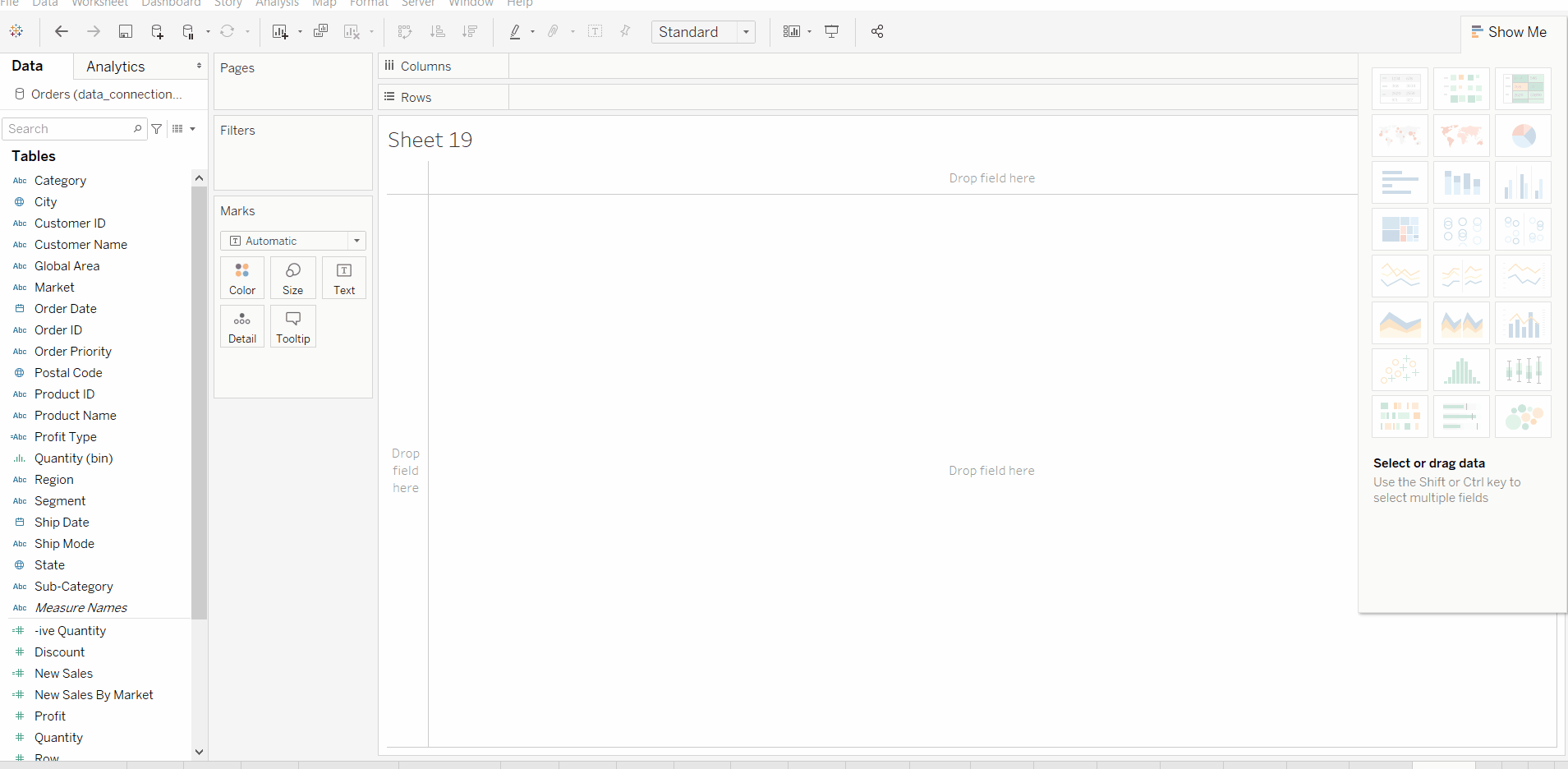OR Function: The Tableau OR function is like an either-or statement in English. If both the conditions are False, Tableau or will return False; otherwise, it returns True. The syntax of this Tableau OR Function is:

```Expression_1 OR Expression_2
```
• To demonstrate this logical function in Tableau, we have to use Calculated Fields.
• To create a calculated field, please navigate to Analysis Tab and choose the Create Calculated Field… option.
• Use the expression in the newly created field.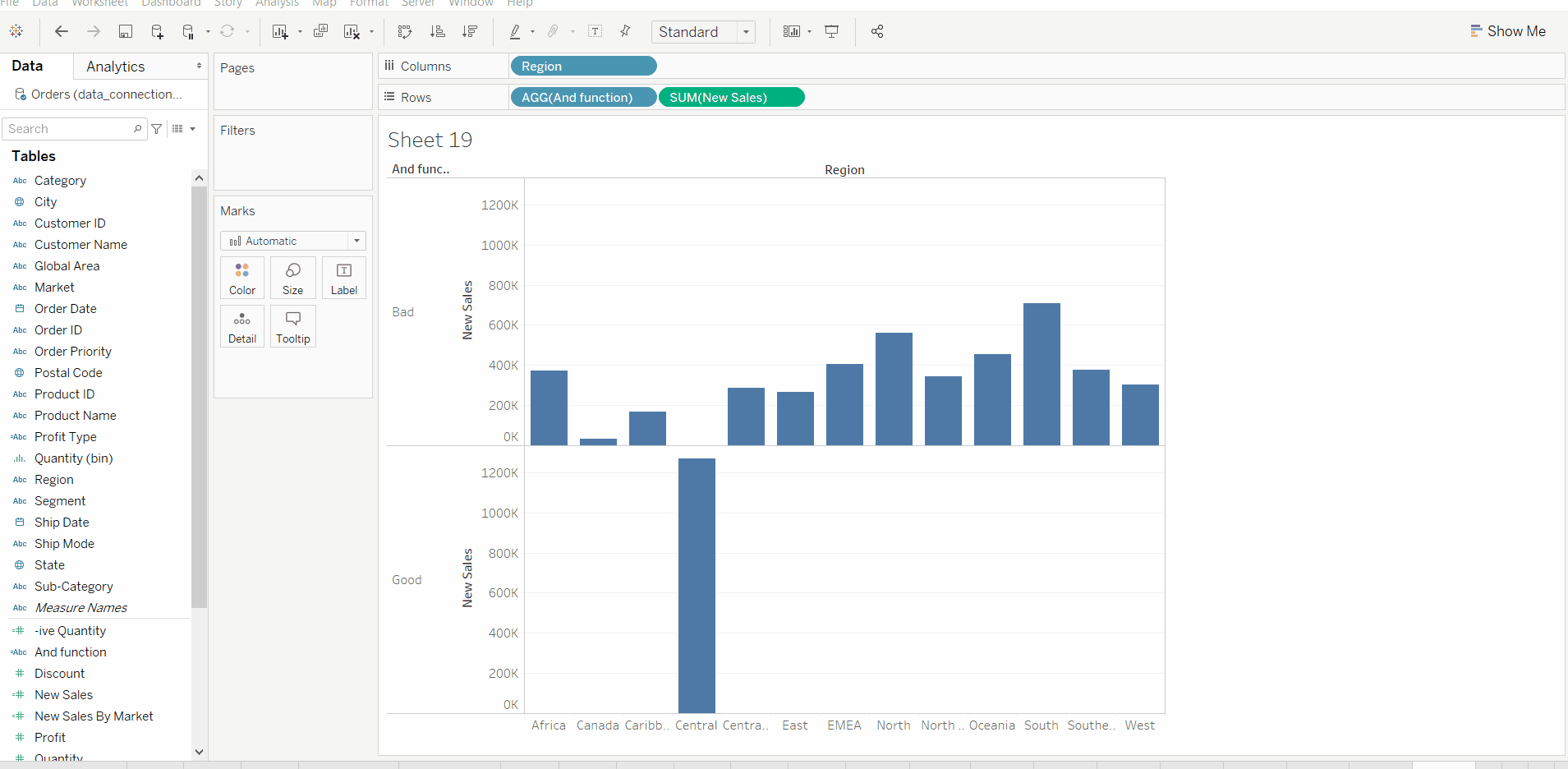IIF Function: The Tableau IIF function is that the simple version of the If Else Function. If both the condition is True, then it’ll return First Statement otherwise, the second statement. The syntax of this Tableau IIF Function is:

```IIF(Expression, True_statement, False_Statement)
```
• To demonstrate this logical function in Tableau, we have to use Calculated Fields.
• To create a calculated field, please navigate to Analysis Tab and choose the Create Calculated Field… option.
• Use the expression in the newly created field.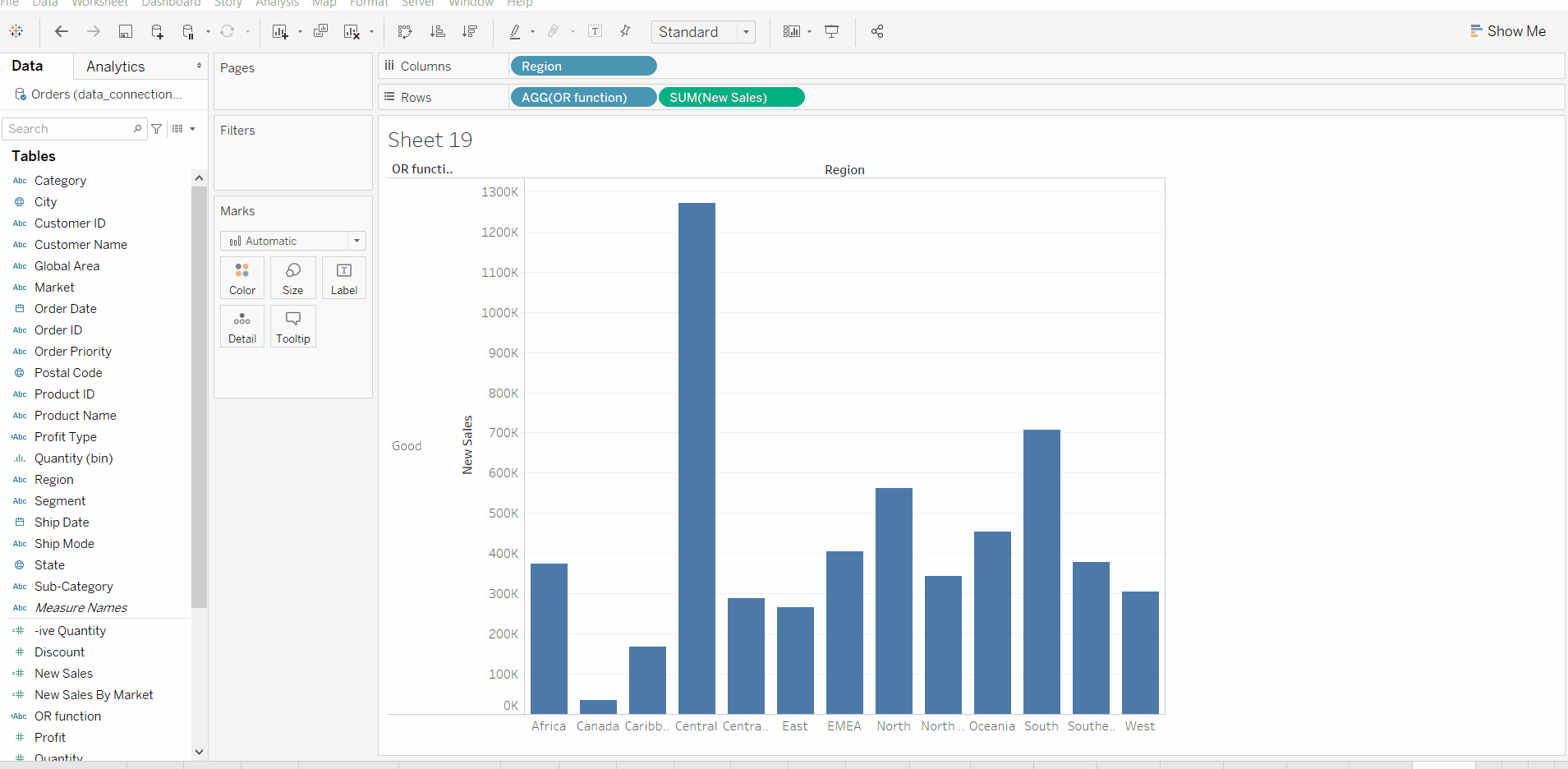NOT Function: The Tableau NOT function return the exact opposite. I mean, True will become false and vice versa. The syntax of this Tableau NOT Function is:

```NOT(Expression)
```
• To demonstrate this logical function in Tableau, we have to use Calculated Fields.
• To create a calculated field, please navigate to Analysis Tab and choose the Create Calculated Field… option.
• Use the expression in the newly created field.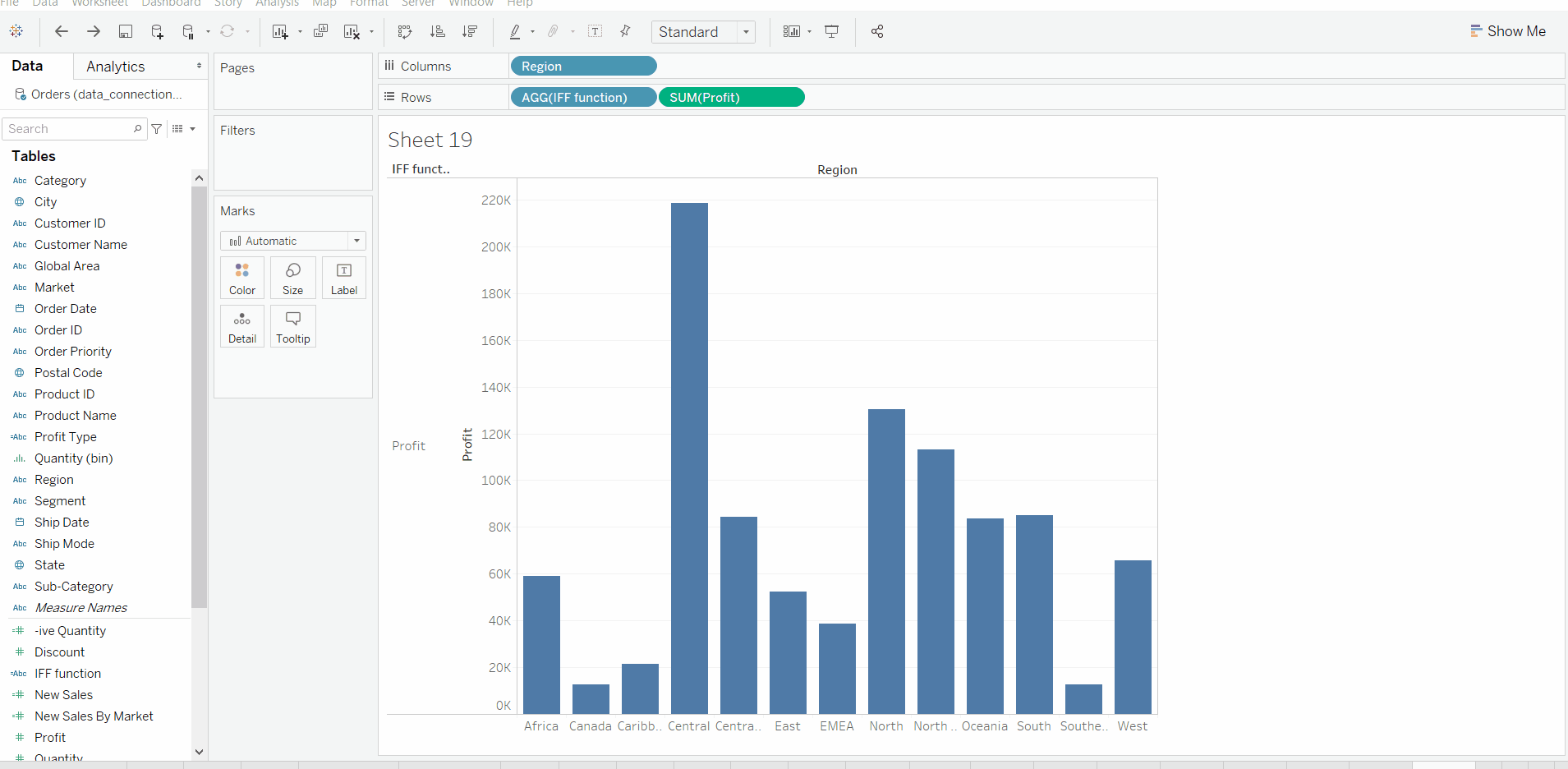ISNULL Function: Tableau ISNULL function will check whether it is NULL or Not. If it’s NULL, then it returns TRUE; otherwise, False will return. The syntax of the Tableau ISNULL Function is:

```ISNULL(Expression)
```
• To demonstrate this logical function in Tableau, we have to use Calculated Fields.
• To create a calculated field, please navigate to Analysis Tab and choose the Create Calculated Field… option.
• Use the expression in the newly created field.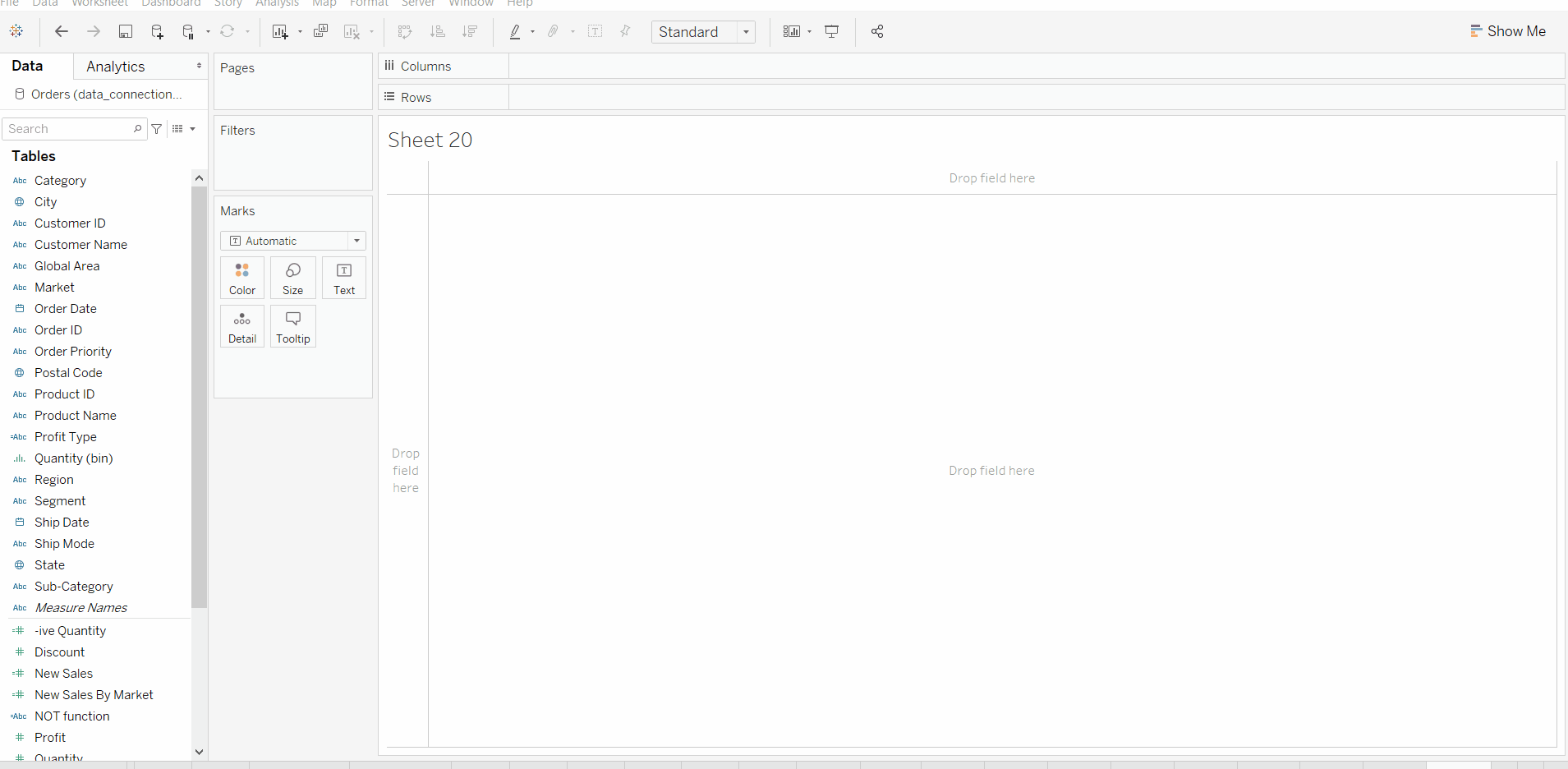ZN Function: Tableau ZN function will return the first values of Not Null values, and 0 for Null values. In simple English, ZN in Tableau is employed to exchange the NULL values with 0. The syntax of the Tableau ZN Function is:

```ZN(Expression)
```
• To demonstrate this logical function in Tableau, we have to use Calculated Fields.
• To create a calculated field, please navigate to Analysis Tab and choose the Create Calculated Field… option.
• Use the expression in the newly created field.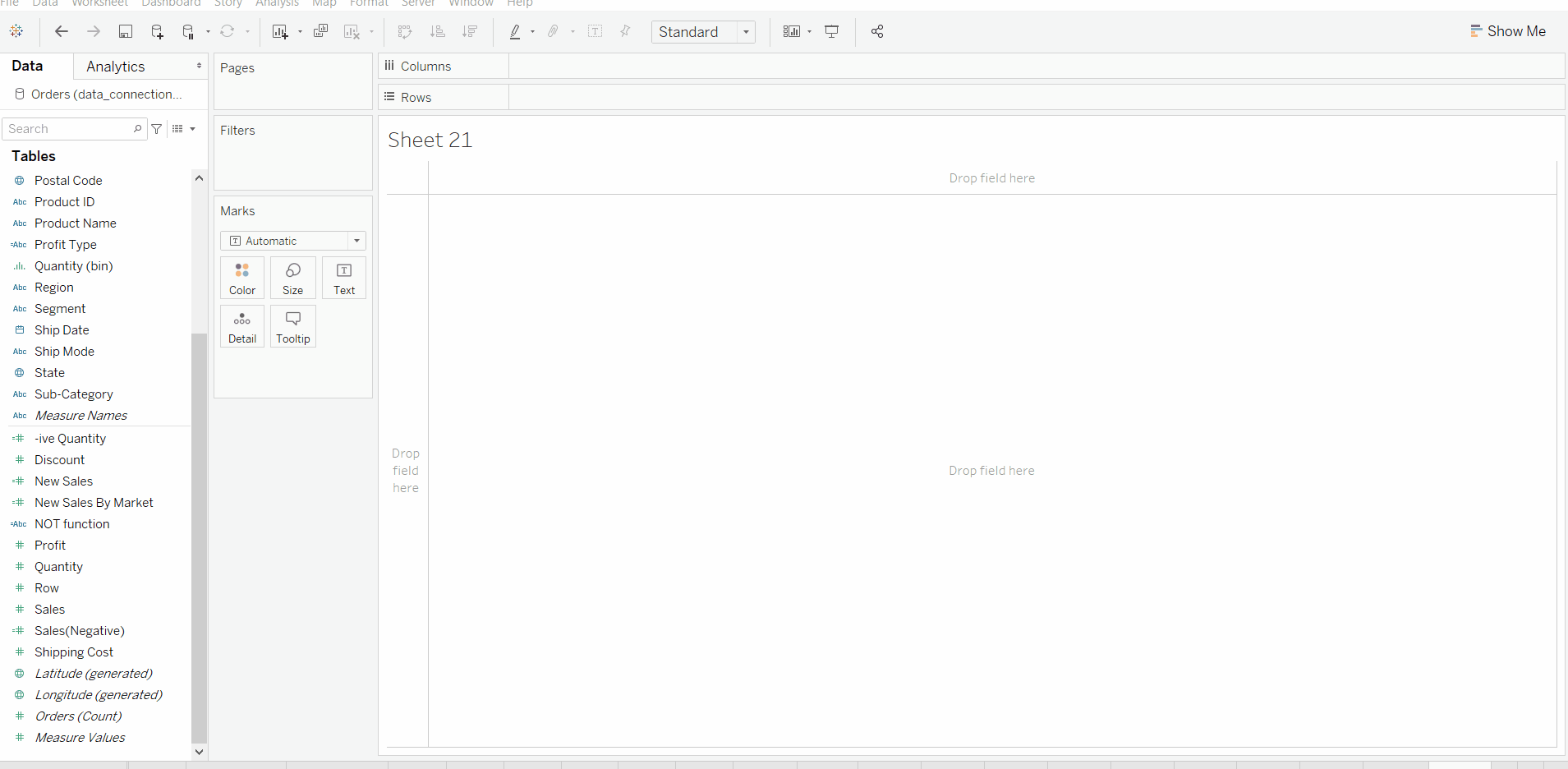IFNULL Function: Tableau IFNULL function is employed to exchange the NULL values together with your own. The syntax of the Tableau IFNULL Function is:

```IFNULL(Expression, Value)
```
• To demonstrate this logical function in Tableau, we have to use Calculated Fields.
• To create a calculated field, please navigate to Analysis Tab and choose the Create Calculated Field… option.
• Use the expression in the newly created field.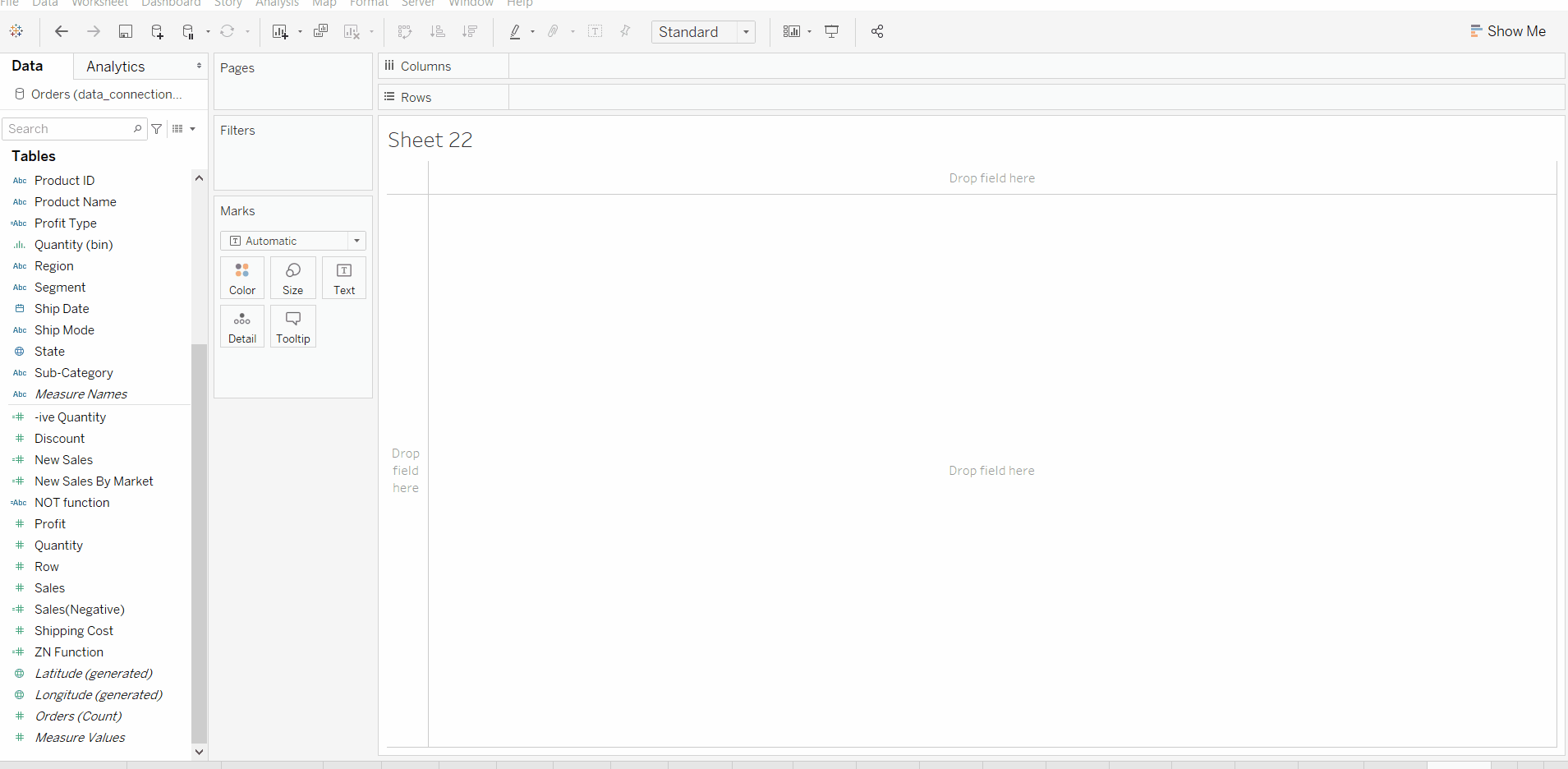IF Function: Tableau If Function is one of the foremost useful decision-making functions. If the function tests the condition and depending upon the condition result, it’ll return the output.

```IF <Expression1> THEN <Statement1>
ELSEIF <Expression2> THEN <Statement2>
ELSEIF <Expression3> THEN <Statement3>
.....
ELSEIF <ExpressionN> THEN <StatementN>
ELSE <Statement>
END
```

IF-END

• In this example, we simply create a new calculated field by using the IF function on a field.
• View new calculated field.
• Use in Visualization.
• It has a drawback that creates null values in case of the false condition.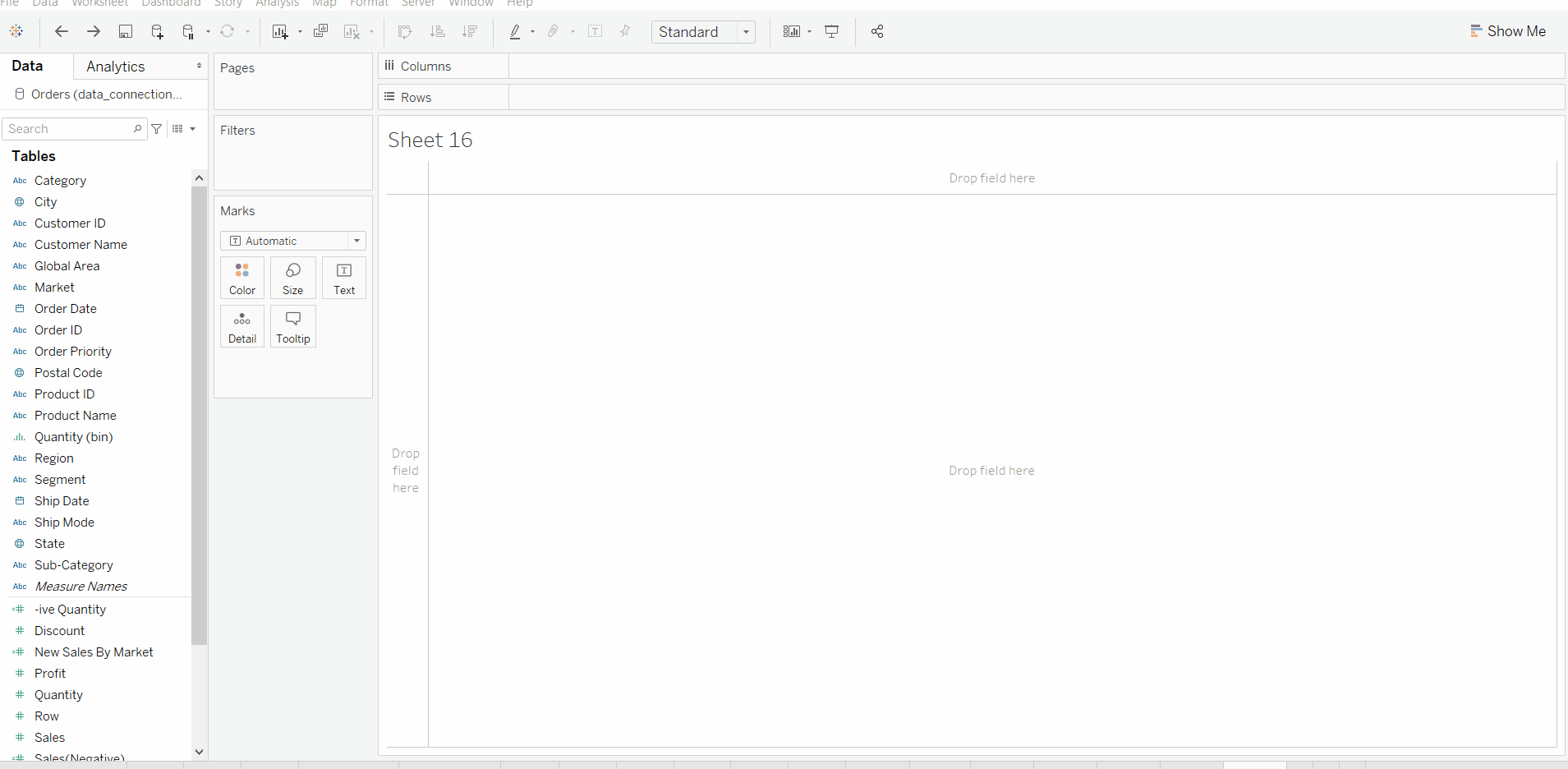IF-ELSE-END

• In this example, we simply edit that previously calculated field by using the IF-ELSE function in the same field.
• View a new calculated field.
• Use in Visualization.
• It overcomes a drawback that creates null values in case of the false condition.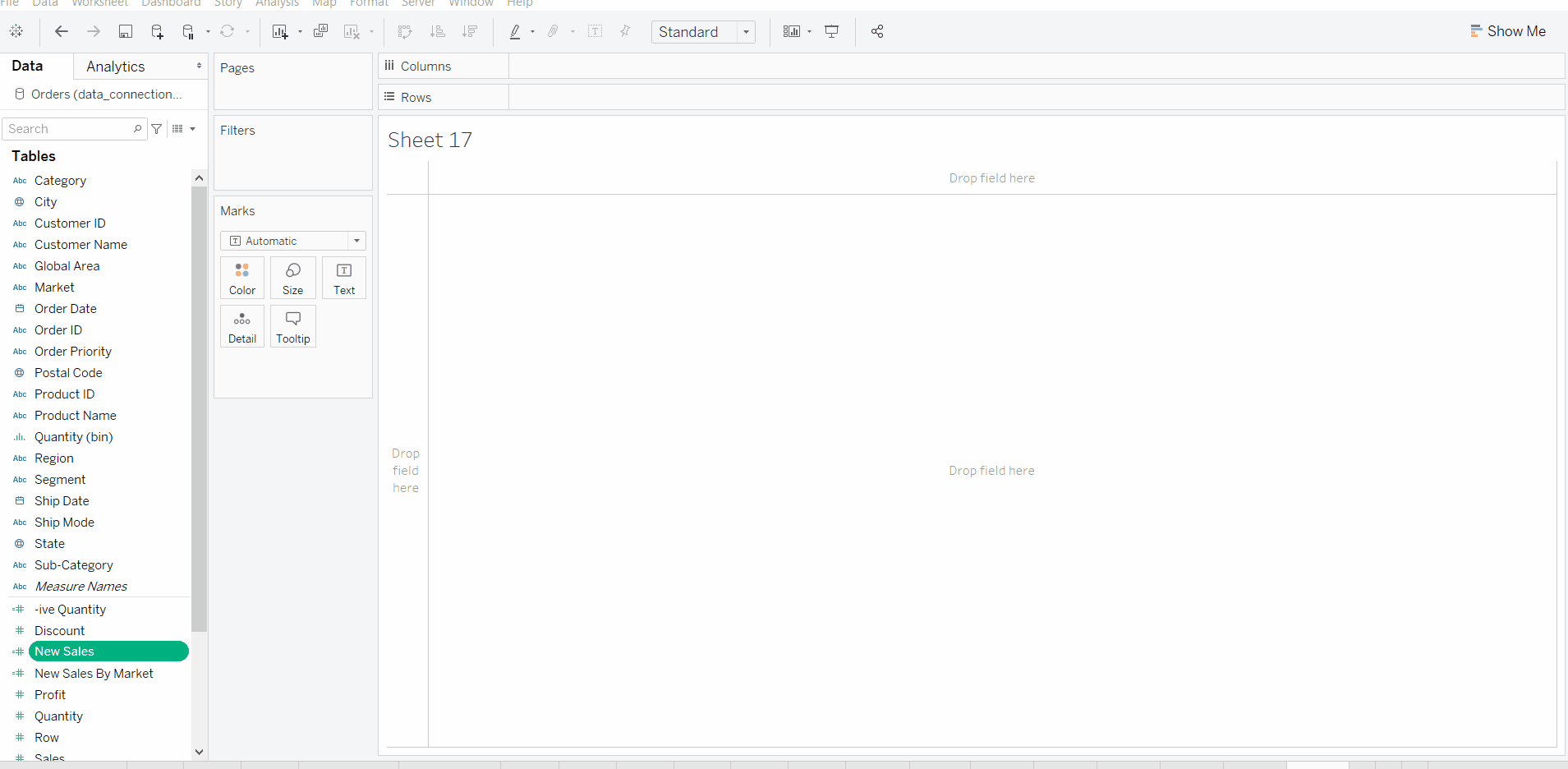IF-ELSEIF-ELSE-END

• In this example, we simply create a new calculated field by using the IF-ELSEIF-ELSE function on a field.
• View a new calculated field.
• Use in Visualization.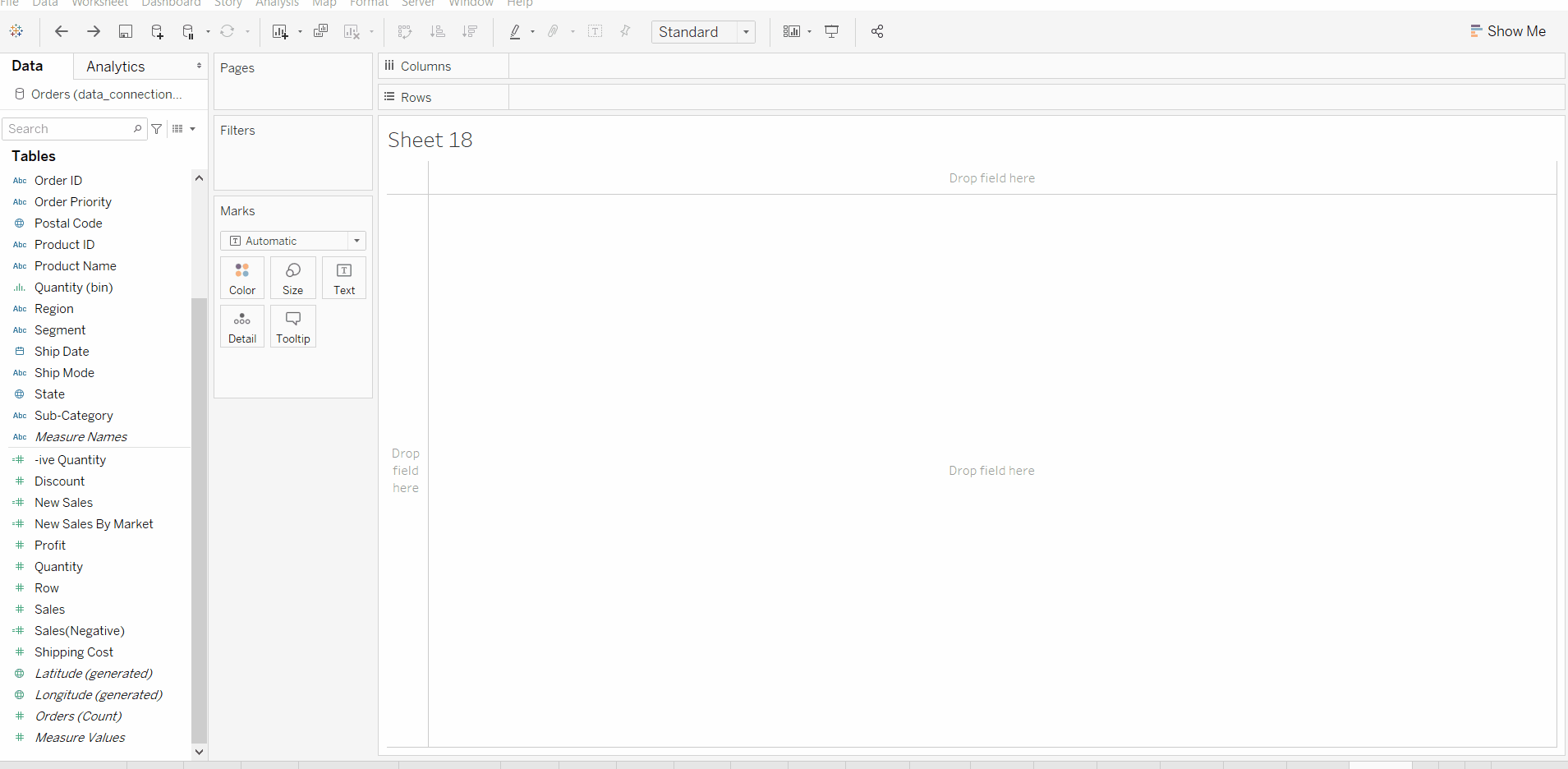Case Function: Case Function is the part of Logical functions in Tableau. These functions are used to perform the logical test and return the required value when the test expression is true.

```CASE [<expression>]
WHEN <expression> THEN <expression>
WHEN <expression> THEN <expression>
ELSE <expression>
END
```
• To demonstrate this logical function in Tableau, we have to use Calculated Fields.
• To create a calculated field, please navigate to Analysis Tab and choose the Create Calculated Field… option.
• Use the expression in the newly created field.